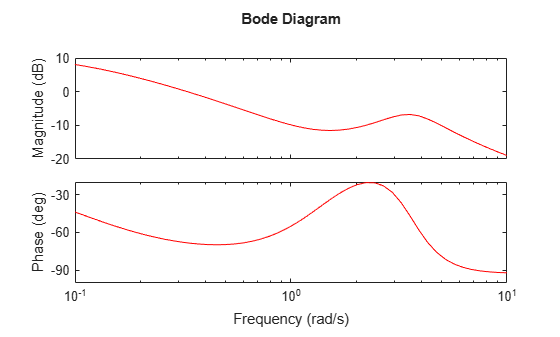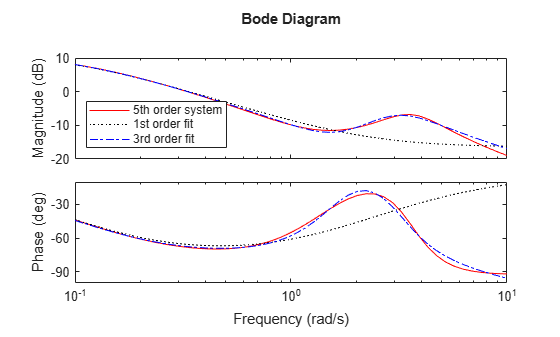fitfrd

Fit frequency response data with state-space model

Syntax

B = fitfrd(A,N)
B = fitfrd(A,N,RD)
B = fitfrd(A,N,RD,WT)

Description

B = fitfrd(A,N) is a state-space object with state dimension N, where A is an frd object and N is a nonnegative integer. The frequency response of B closely matches the D-scale frequency response data in A.

A must have either 1 row or 1 column, although it need not be 1-by-1. B will be the same size as A. In all cases, N should be a nonnegative scalar.

B = fitfrd(A,N,RD) forces the relative degree of B to be RD. RD must be a nonnegative integer. The default value for RD is 0. If A is a row (or column) then RD can be a vector of the same size as well, specifying the relative degree of each entry of B. If RD is a scalar, then it specifies the relative degree for all entries of B. You can specify the default value for RD by setting RD to an empty matrix.

B = fitfrd(A,N,RD,WT) uses the magnitude of WT to weight the optimization fit criteria. WT can be a double, ss or frd. If WT is a scalar, then it is used to weight all entries of the error criteria (A-B). If WT is a vector, it must be the same size as A, and each individual entry of WT acts as a weighting function on the corresponding entry of (A-B).

Examples

collapse all

Use the fitfrd command to fit D-scale data.

Create D-scale frequency response data from a fifth-order system.

sys = tf([1 2 2],[1 2.5 1.5])*tf(1,[1 0.1]);
sys = sys*tf([1 3.75 3.5],[1 2.5 13]);
omeg = logspace(-1,1);
sysg = frd(sys,omeg);
bode(sysg,'r-');You can try to fit the frequency response D-scale data sysg with a first-order system, b1. Similarly, you can fit the D-scale data with a third-order system, b3.

b1 = fitfrd(sysg,1);
b3 = fitfrd(sysg,3);

Compare the original D-scale data sysg with the frequency responses of the first and third-order models calculated by fitfrd.

b1g = frd(b1,omeg);
b3g = frd(b3,omeg);
bode(sysg,'r-',b1g,'k:',b3g,'b-.')
legend('5th order system','1st order fit','3rd order fit','Location','Southwest')Limitations

Numerical conditioning problems arise if the state order of the fit N is selected to be higher than required by the dynamics of A.